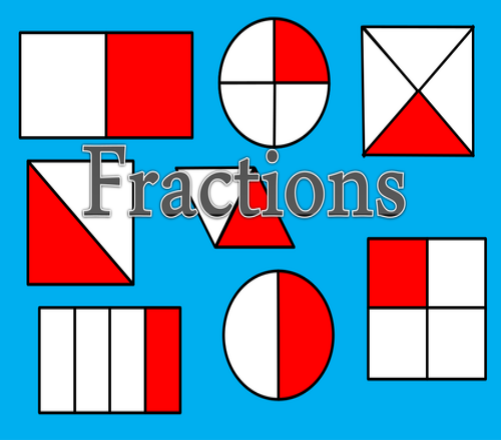# How Well Do You Know Fractions Of Shapes?

10 Questions | Total Attempts: 204SettingsRepresenting data in a fraction or ratio format has always been the simple solution to represent numbers of equal parts or cannot be represented clearly to decimal places, take a quiz and see what you know about using shapes to represent fractional figures.

• 1.
In a circle in which is broken into for places, three-part are painted in blue and a part is left empty, how is this represented in a fraction form?
• A.

½

• B.

¾

• C.

¼

• D.

2/3

• 2.
A rectangle object comprises of six parts, three parts were filled with objects while the other parts were left empty, represent this in fraction form?
• A.

3/6

• B.

1/6

• C.

3/6

• D.

3/3

• 3.
A triangle was broken into 3 parts equally with a section filled and other two section left empty, what is the fractional representation?
• A.

3/3

• B.

2/4

• C.

½

• D.

1/3

• 4.
A balloon ball was filled up with paint after it was broken into six parts, only one part was filled up while others were empty, represent this in fractional form?
• A.

6/6

• B.

5/6

• C.

2/6

• D.

1/6

• 5.
In representing fraction as a mathematical form, there are two figures involved with a line in-between them, what is the upper figure called?
• A.

Division

• B.

Fraction

• C.

Numerator

• D.

Denominator

• 6.
In representing fraction as a mathematical form, there is two figure involve with a line in-between them, what is the lower figure called?
• A.

Denominator

• B.

Ratio

• C.

Division

• D.

Numerator

• 7.
The fraction can only be represented in a numerator and denominator format, it can also be represented in a ratio format, 3/4 in a ration format is?
• A.

3|4

• B.

3:4

• C.

3.4

• D.

3;4

• 8.
In mathematics, the set of all numbers that can be expressed in the form a/b, where a and b are integers and b is not zero, is called the set of rational numbers and is represented by the symbol Q which stands for?
• A.

Qformular

• B.

Quora

• C.

Quotient

• D.

Query

• 9.
If you brake a circle into 4 sections, and then painted two third of the circle, you are left with how many empty sections?
• A.

3

• B.

4

• C.

1

• D.

2

• 10.
If you are given a box partitioned into 4 parts, you are then asked to shade a part of the represent the result in a fraction form
• A.

¾

• B.

4/4

• C.

2/4

• D.

‘¼

Related TopicsBack to top